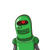# Which term of the AP:3, 15, 27, 39…. will be 132 more than its 54th term?​

Which term of the AP:3, 15, 27, 39…. will be 132 more than its 54th term?

### 1 thought on “<br />Which term of the AP:3, 15, 27, 39…. will be 132 more than its 54th term?<br />​”

1.## 65th term

### Step-by-step explanation:

AP:3, 15, 27, 39….

a = 3 , d = 15 – 3 = 12 ,  n = 54

an = a + d(n – 1)

an = 3 + 12(54 – 1)

= 3 + (12 * 53)

= 3 + 636

an = 639

am = 639 + 132

am = 771

am = a + d(m – 1)

771 = 3 + 12(m – 1)

771 – 3 = 12(m – 1)

12(m – 1) = 768

m – 1 = 768 / 12

m – 1 = 64

m = 65

∴ 65th term is  132 more than its 54th term